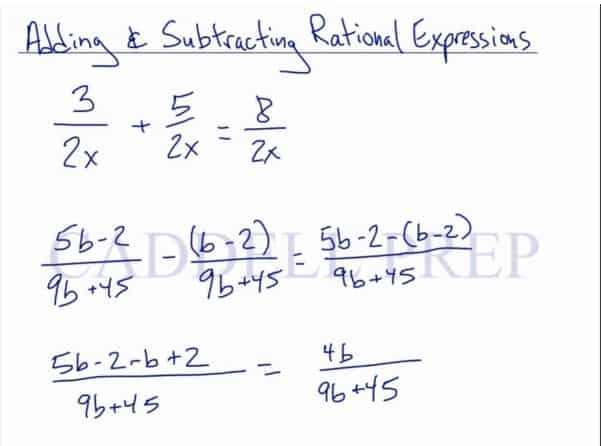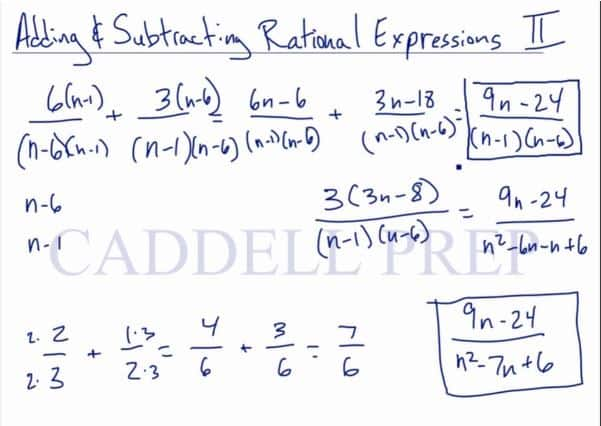Part 1

In the first video, we are going to add and subtract rational expressions. After you finish this lesson, view all of our Algebra 1 lessons and practice problems.

For example:$\dfrac{3}{2x}+\dfrac{5}{2x}$

Simply combine the numerators together and keep the denominator if the denominators are the same, to get:$\dfrac{8}{2x}$

Let’s look at another example:$\dfrac{2}{6n}+\dfrac{4}{2mn}$

Since the denominators are not the same, multiple each fraction to achieve the least common multiple$\dfrac{(m)2}{(m)6n}+\dfrac{(3)4}{(3)2mn}$$\dfrac{2m}{6mn}+\dfrac{12}{6mn}$

Now, we can add the terms together$\dfrac{2m+12}{6mn}$

In order to reduce the expression, factor if necessary$\dfrac{2(m+6)}{6mn}$

Reduce, and our final answer is$\dfrac{(m+6)}{3mn}$

Video-Lesson Transcript

Let’s go over how to add and subtract rational expressions.

We have two rational expressions here$\dfrac{3}{2x} + \dfrac{5}{2x}$

Just like any fractions that need to add or subtract, we have to have a common denominator.

In this case, we do.

So, we have the same denominator and then just add the numerator.$= \dfrac{8}{2x}$

Let’s look at a more difficult one.$\dfrac{5b - 2}{9b + 45} - \dfrac{b - 2}{9b + 45}$

Again, we have common denominators. So we’ll just copy it.

We’ll subtract the numerator as necessary.$= \dfrac{5b - 2 - (b - 2)}{9b + 45}$

Then distribute the negative sign$= \dfrac{5b - 2 - b + 2}{9b + 45}$

Then, let’s combine the like terms$= \dfrac{4b}{9b + 45}$Let’s look at an example where we don’t have common denominators.$\dfrac{2}{6n} + \dfrac{4}{2mn}$

What we want to do first is to write this with common denominators.

So, we need the least common multiple of the two denominators.${6n}$ and$2mn$

What can we multiply these by to give us something that will equal each other?

Let’s look at the numbers first and identify the least common multiple.${6n}$ will be$6mn$,$2mn$ will be$6mn$

We multiplied$6n$ by$m$ and$2mn$ by$3$.

So here’s our solution:$\dfrac{(m) 2}{(m) 6n} + \dfrac{(3) 4}{(3) 2mn}$$= \dfrac{2m}{6mn} + \dfrac{12}{6mn}$

Now we can add these together.$= \dfrac{2m + 12}{6mn}$

Let’s look at it further.

We can still reduce this since there’s a factor$= \dfrac{2 (m + 6)}{6mn}$$= \dfrac{m + 6}{3mn}$

Part 2

In the second video, we are going to add and subtract rational expressions that are more complex.

For example:$\frac{6}{n-6}+\frac{3}{n-1}$

Multiply each fraction to achieve the least common multiple$\frac{(n-1)6}{(n-1)(n-6)}+\frac{(n-6)3}{(n-6)(n-1)}$

Use the distributive property to multiply$\frac{6n-6}{(n-1)(n-6)}+\frac{3n-8}{(n-1)(n-6)}$

Now that we have common denominators, add the numerators together$\frac{9n-24}{(n-1)(n-6)}$

Factor any expression if necessary$\frac{3(3n-8)}{(n-1)(n-6)}$

Since nothing can be reduced, we are going back to the original expression$\frac{9n-24}{(n-1)(n-6)}$

Use FOIL (First, Outside, Inside, Last) to multiply$\frac{9n-24}{n^2-6n-n+6}$

Combine like terms, and we have$\frac{9n-24}{n^2-7n+6}$

Examples of Adding And Subtracting Rational Expressions

Example 1$\dfrac{u-v}{8v}+\dfrac{6u-3v}{8v}$
Since we have the same denominator, just add the numerator.$\dfrac{u-v+6u-3v}{8v}$$\dfrac{7u-4v}{8v}$

Example 2$\dfrac{x-1}{x+1}+\dfrac{3}{x}$

Since the denominators are not the same, multiple each fraction to achieve the least common multiple$\dfrac{x(x-2)}{x(x+1)}+\dfrac{3(x+1)}{x(x+1)}$$\dfrac{x^2-2x}{x^2-x}+\dfrac{3x+3}{x^2-x}$

Now, we can add the terms together$\dfrac{x^2-2x+3x+3}{x^2-x}$$\dfrac{x^2+x+3}{x^2-x}$

Video-Lesson Transcript

Let’s go over how to add and subtract rational expressions part 2.

We’re going to focus on binomials on the denominator.

For example:$\dfrac{6}{n - 6} + \dfrac{3}{n - 1}$

First, we want to write this with common denominators.

What could be the least common multiple of these two?$n - 6$,$n - 1$

Let’s take a step back and take a look at adding fractions.

If we have$\dfrac{2}{3} + \dfrac{1}{2}$

what is the least common multiple of$3$ and$2$?

It’s$6$.

We got that by simply multiplying the two numbers.

Now, we’ll have$= \dfrac{(2) 2}{(2) 3} + \dfrac{(3) 1}{(3) 2}$$= \dfrac{4}{6} + \dfrac{3}{6}$$= \dfrac{7}{6}$

So, we’ll do the same thing here.

Let’s just multiply these together.$\dfrac{6}{n - 6} + \dfrac{3}{n - 1}$$= \dfrac{6 (n - 1)}{(n - 6) (n - 1)} + \dfrac{3 (n - 6)}{(n - 1) (n - 6)}$$= \dfrac{6n - 6}{(n - 1) (n - 6)} + \dfrac{3n - 18}{(n - 1) (n - 6)}$

Now, let’s add the numerators together.$= \dfrac{9n - 24}{(n - 6) (n - 1)}$

The reason why we didn’t multiply the denominators together is because it’s a lot easier to factor this way.

Now, we can factor the numerator only and keep the denominator as it is.$= \dfrac{3 (3n - 8)}{(n - 6) (n - 1)}$

But there isn’t anything to cancel out.

So, let’s just solve it.$= \dfrac{9n - 24}{n^2 - 7n + 6}$

This format is also acceptable:$= \dfrac{9n - 24}{(n - 6) (n - 1)}$Let’s look at this one$\dfrac{3}{n - 4} + \dfrac{2n - 8}{n^2 - n + 12}$

So, we’ll just multiply the denominators together to get the common denominator.

This will be a bit more complicated because we’re going to multiply a binomial to a trinomial.

Multiplying these is going to give us a big expression. If we just multiply them upfront.$= \dfrac{3 (n^2 - n - 12)}{n - 4 (n^2 - n - 12)} + \dfrac{2n - 8 (n - 4)}{n^2 - n + 12 (n - 4)}$

This is going to be confusing.

We’ll still get the correct answer but this is a lot of work.

So, let’s look at another option.

What we can do is to look at the factors of these denominators.$n - 4$ stays as is

Let’s factor$n^2 - n - 12$ out$= (n - 4) (n + 3)$

The first denominator is already$n - 4$, so we’ll just multiply it by$n + 3$.

At this point, we already have common denominators.$= \dfrac{3 (n + 3)}{n - 4 (n + 3)} + \dfrac{2n - 8 }{(n - 4) (n + 3)}$

The numerator for the second expression stays the same because all we did was just to factor its denominator.

The denominator didn’t change in the second expression.$= \dfrac{3n + 9}{n - 4 (n + 3)} + \dfrac{2n - 8 }{(n - 4) (n + 3)}$

We have like terms here so let’s combine.$= \dfrac{5n + 1}{(n - 4) (n + 3)}$